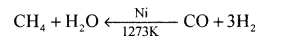# 1st PUC Chemistry Previous Year Question Paper March 2019 (North)

Students can Download 1st PUC Chemistry Previous Year Question Paper March 2019 (North), Karnataka 1st PUC Chemistry Model Question Papers with Answers helps you to revise the complete Karnataka State Board Syllabus and score more marks in your examinations.

## Karnataka 1st PUC Chemistry Previous Year Question Paper March 2019 (North)

Time: 3.15 Hours
Max Marks: 70

Instruction:

1. The questions paper has five parts A, B, C, D and E. All parts are compulsory.
2. Write balanced chemical equations and draw labeled diagram wherever allowed.
3. Use log tables and simple calculations f necessary (use of scientific calculations is not allowed).

Part – A

I. Answer ALL of the following (each question carries one mark): ( 10 × 1 = 10 )

Question 1.
What is the S.I. unit of Electric Current?
ampere (or) A.

Question 2.
State Dalton’s law of Partial Pressure.
“The total pressure exerted by a mixture of non-reacting gases is equal to the sum of the partial pressures of all the component gases at constant temperature.”

Question 3.
Write the conjugate base for HCO3.
CO3-2.Question 4.
Write the valence shell electronic configuration of P-block elements.
nS2.p1-6.

Question 5.
Assign the oxidation number of ‘Mn’ in MnO4.
+7.

Question 6.
Name the alkali metal which has high hydration enthalpy.
Lithium.

Question 7.
Give the chemical formula of Borax.
Na2B4O7 10H2O.

Question 8.
What are silicones?
Polymeric organo-silicon compounds containing Si-O-Si bonds are called silicones.Question 9.
Write IUPAC name of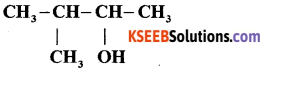4 methyl-butan-2-ol.

Question 10.
Name the organic product obtained when Benzene is treated with excess of chlorine in presence of anhydrous Aluminium chloride.
Chlorobenzene.

Part – B

II. Answer any FIVE of the following questions carrying TWO marks: ( 5 × 2 = 10 )

Question 11.
A solution is prepared by adding 2g of a substance A to 18 g of water. Calculate the mass percent of the solute.Question 12.
Derive the relation between Density and Molar mass of a gaseous substance from ideal gas equation.
W.K.T PV =- nRTWhere ρ = density, P = Pressure of a gas, M = Molar Mass of gas,
R = gas constant, T = Kelvin Temperature.Question 13.
Write the resonance structures of ozone.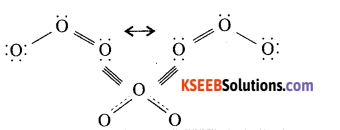Question 14.
What happens when
(i) Quicklime is heated with silica.
(ii) Sodium burns vigorously in oxygen.
(i) Calcium silicate is formed. CaO + SiO2 → CaSiO3
(ii) Sodium bums vigorously in oxygen.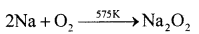Question 15.
Diamond is covalent, yet it has high melting point. Why?
Due to strong covalent bonds holding all the carbon atoms in diamond together.

Question 16.
How do you prepare Benzene from Ethyne.
When ethyne is passed through a red hot copper tube, it polymerises to form benzene.Question 17.
Explain the Friedel crafts alkylation of Benzene with the equation.
When Benzene is treated with an alkylhaiide in presence of anhydrous Aluminium Chloride, alkylation takes place to give alkyl benzene.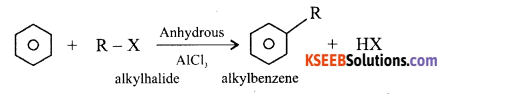Question 18.
What are particulate pollutants? Give an example.
Particulate pollutants are solid particles in the air. Example: dust, fumes, mist, smoke.Part – C

III. Answer any FIVE of the following questions carrying THREE marks: ( 5 × 3 = 15 )

Question 19.
(a) Define Electro negativity. How does it vary along a group?
The ability of an atom to attract the shared electron pair (of a covalent bond) in a molecule towards itself is called electro negativity. In a period from left to right the electro negativity increases. Down a group electronegativity value decreases.

(b) Which of the following will have most negative electron gain enthalpy?
P, S, Cl, F
F.

Question 20.
Explain SP-hybridisation in BeCl2 molecule.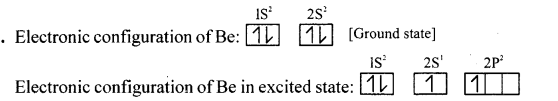One 2S and one 2P orbital of Beryllium undergoes SP hybridisation giving rise to two SP hybrid orbitals of same energy. Which has a linear structure. Two SP hybrid orbitals of Beryllium overlaps with 2P orbital of Chlorine to form two Be-Cl covalent bonds with a bond angle of 180°.Question 21.
(a) Write any two differences between sigma-bond and Pi-bond.
(b) Name the type of Hydrogen bonding in ortho-nitrophenol.
(a)
σ bond :

1. Overlapping is along the axis
2. Overlapping is maximum
3. Due to maximum overlap the bond is strong
4. s-orbital can participate

π bond :

1. Overlapping is on side wise
2. Overlapping is minimum
3. Due to minimum overlap, the band is weak
4. s-orbital cannot participate

(b) Intramolecular hydrogen bonding.

Question 22.
Write the Electronic configuration of Oxygen molecule. Predict its magnetic property and calculate its Bond order.
Atomic number of oxygen is 8: 1s2 2s2, 2P4(a) Bond order = $$\frac{8-4}{2}$$ = 2
(b) Electronic configuration of oxygen molecule is
KKσ2s2 σ*2s2 σ2pz2 π2px1 π2p2y π*2p1x π*2p1y
(c) Magnetic property: Paramagnetic.

Question 23.
Balance the Redox-reaction by using Oxidation number method in acidic medium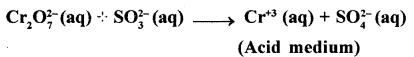Cr2O2-7 + SO32- → Cr3++ SO4-2 (Acidic medium)
Step 1.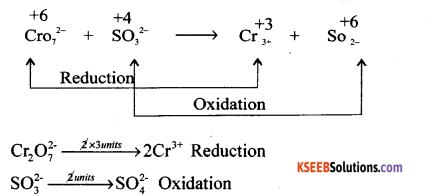Multiply reduction equation by 1 unit and oxidation equation by 3 units
Cr2O2-7 → 2Cr3+
3SO32- → 3SO4-2
Adding both equations Cr2O2-7 + 3SO32- → 2Cr3+ + 3SO42-
since there are 4 oxygen atom more on LHS, 4H2O is added on RHS. The equation is balanced WRT H+ ion.
Cr2O2-7 + 3SO32- + 8H+ → 2Cr3+ + 3SO42- + 4H2O

Question 24.
(a) How temporary Hardness of water is removed by Boiling?
Hard water can be softened by the following methods depending upon the nature of hardness. Temporary hardness: (i) By boiling: It can be removed by merely boiling the water. Boiling decomposes the bicarbonates to give carbon dioxide and insoluble carbonates, which can be removed by filtration.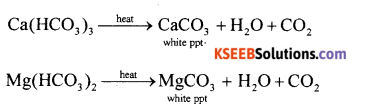(b) What is the composition of water gas?
CO + H2

Question 25.
(a) Complete the following reactions.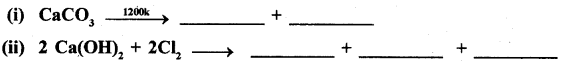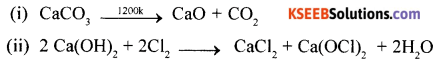(b) Lithium shows diagonal relationship with Magnesium. Give reason.
Small size and high charge density.Question 26.
(a) Write the chemical formula of Inorganic benzene.
B3N3H6.

(b) Write the dimeric structure of Aluminium chloride.(c) Define catenation.
Carbon atoms have a remarkable property of joining with one another in a large number to form a long chain and rings. This property is known as catenation or self linkage.

Part – D

IV. Answer any FIVE of the following questions carrying FIVE marks: ( 5 × 5 = 25 )

Question 27.
(a) Calculate the amount of carbon dioxide produced by the combustion of 24g of methane.(b) Define Mole. What is the value of Avagadro’s Number.
Mass of a substance which contains avogadro number of particles.
Avogadro number = 6.023 x 1023

(c) Express the number 232.508 in scientific notation.
232.51.

Question 28.
(a) What are the observations made in Rutherford a-particle experiment.
The observations of p ray scattering experiments:

1. About 99% of α particles are simply passed through the gold leaf as if they did not come across any obstruction in their path.
2. A small number of them got deflected by small angles.
3. About 1 out of 20,000 α particles got deflected by more than 90°. A few of them even retracted their path.

(b) Give any two postulates of Bohr’s theory of Atomic model.
Bohr’s model of an atom, the postulates are:

1. Electrons revolve around the nucleus of an atom in a certain definite path called orbit or stationary state of shell.
2. The shells are having different energy levels denoted as K, L, M, N…
3. As long as the electron remains in an orbit, they neither absorb nor emit energy.
4. The electron can move only in that orbit in which angular momentum is quantized,
i.e., the angular momentum of the electron is an integral multiple of $$\frac{h}{2 \pi}$$

Question 29.
(a) Name any three quantum numbers and write their significance.
(i) Principal Quantum number : Determines energy and size of the orbital.
(n)
(ii) Azimuthal Quantum number : Determines the shape of the orbitals (sub-shells).
(l)
(iii) Magnetic Quantum number : Determines the orientation of the orbitals.
(m)
(iv) Spin Quantum number : Determines the spin motion of the orbitals.
(s)

(b) State heisenberg’s uncertainty principle. Write its mathematical equation.
It is impossible to determine both the momentum (particle nature) and position (wave nature) of a moving sub atomic particle simultaneously with absolute accuracy.
Mathematically ∆x × ∆p = h / 4π where Ax = uncertainty in position:
∆p = uncertainty in momentum; h = Plank’s constant = 6.626 × 10-34 Js.Question 30.
(a) Write any three postulates of Kinetic theory of gases.

1. Gases are made up of large number of the minute particles.
2. Pressure is exerted by a gas.
3. There is no loss of kinetic theory.
4. Molecules of gas attract on one another.

(b) Define critical temperature. Write the value of critical temperature for CO2.
The maximum temperature at which a gas can be converted into liquid by applying pressure.
Critical temperature of C2→ 30.98°C.

Question 31.
(a) Calculate the standard enthalpy of formation of Methane. Given that the standard enthalpy of combustion of Methane, Carbon and Hydrogen are -890.3 kJ,
C(S) + 2H2(g) → CH4(g) ∆H ….(1)
C(S) + O2 (g) → CO2(g) ∆H = -393.5kJ ….. (2)
H2(g) + 1/2 O2 (g) → H2O() ∆H = -285.8kJ …… (3)
CH4(g) + 2O2 (g) → CO2(g) + 2H2O(I) ∆H = -890.3kJ ….. (4)
Eqn. (2) × , Eqn. (3) × 2, reverse Eqn. (4), add
H2(g) + O2(g) → 2H2O(l) ∆H = -571.6kJ
CO2(g) + 2H2O(l) → CH4(g) + 20,(g) ∆H = +890.3kJ
C(S) + 2H2(g) → CH4(g) ∆H = -74.8kJ

(b) What is the change in the value of entropy when ice melts to give water.
+ve (or) positive.

Question 32.
(a) State Hess’s law of constant heat summation. Illustrate with examples.
Hess’s law : Whether a chemical reaction takes place in a single step or in several steps, the ‘ total change in enthalpy remains the same.
Consider the formation of CO2 from C.

I Method (Single step): C(s) + O2 (g) → CO2(g); ∆H = -xkJ
Heat liberated = xkJ

II Method (Two step) : C(s) + $$\frac{1}{2}$$ O2 (g) → CO(g);∆H = -x,kJ
CO(g) +$$\frac{1}{2}$$ O2(g) → CO2(g) ; ∆H = -x2kJ .
Total heat liberated = (x1 + x2 )kJ
According to Hess’s law, x = x1 + x2

(b) Mention any two thermodynamic criteria for spontaneous process.
Ansewr:
Change in Enthalpy should be positive and Change in Entropy should be positive.

Question 33.
(a) Define Equilibrium constant. Derive an expression for the equilibrium constant Kc for the reaction.
Answre:
Equilibrium constant (Kc) is defined as the ratio of product of equilibrium concentrations of products to the product of equilibrium concentrations of reactants each concentration term being raised to its stoichiometric coefficient.
Consider the following reaction at equilibrium.
aA + bB ⇌ cC + dD
Applying law of mass action
Rf ∝ [A]a[B]b
Rf = Kf[A]a[B]b
Rb ∝ [C]c[D]d
Rb = Kf[C]c[d]d
At equilibrium
Kf[A]a[B]b = Kf[C]c[d]d
KC = $$\frac{\mathrm{K}_{f}}{\mathrm{K}_{b}}=\frac{[\mathrm{C}]^{\mathrm{C}}[\mathrm{D}]^{d}}{[\mathrm{A}]^{\mathrm{a}}[\mathrm{B}]^{\mathrm{b}}}$$

(b) What is the effect of increase in temperature for the reaction?
2NO2(g) ⇌ N2(g)O4(g); ∆H = -572.RJ
Increase in temperature increases the rate of backward reaction because forward reaction is exothermic.

(c) Write the relationship between the solubility and solubility product of AB type salt.
KSp = S2 (or) S = √KSp

Question 34.
(a) Calculate the pH of 0.001 M NaOH. Assuming complete dissociation of base.
[OH ] = 10-3 M pOH = -log[10-3] = 3
pH + pOH = 14 pH = 14 – 3 = 11

(b) Classify the following species into Lewis acid and Lewis base.
(i) HO (ii) BCl3
(i) HO → Lewis base (ii) BCl3 → Lewis acid
(c) Give an example for basic buffer.
NH4Cl + NH4OHPart – E

V. Answer any TWO of the following questions earning FIVE marks: ( 2 x 5 = 10 )

Question 35.
(a) Explain Functional isomerism with an example.
Two or more compounds having same molecular formula but different functional groups are called functional isomers. Ex: C3H6O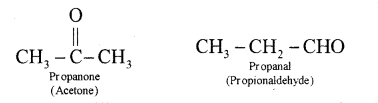(b) For the compound. CHsC-CH = CH CH3
(i) Write the bond line formula for the compound.
(ii) Identify the number of Sigma and Pi-bonds.A free radical can be defined as an atom or group of atoms having an odd or unpaired electron. Putting a dot (•) against the symbol of atom or group of atoms.Question 36.
(a) How do you estimate carbon and hydrogen present in the organic compound by Leibigs method. (Diagram not necessary)
Principle: A known mass of an organic compound is strongly heated with dry cupric oxide (CuO), when carbon and hydrogen are quantitatively oxidized to CO2 and H2O respectively. The masses of CO2 and H2O thus formed are determined. From this, the percentages of carbon and hydrogen can be calculated.

Procedure : Pure and dry oxygen is passed through the entire assembly of the apparatus (Figure) till the CO2 and moisture is completely removed.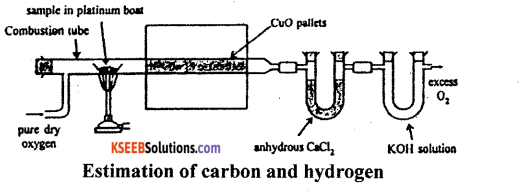A boat containing weighed organic substances is introduced inside from one end of the combustion tube by opening it for a while. The tube is now strongly heated till the whole of the organic compound is burnt up. The flow of oxygen is continued to drive CO2 and water vapours completely to the U-tubes. The apparatus is cooled and the U-tubes are weighed separately.

Observation and calculations:

1. Mass of organic compound taken = w.g.
2. Mass of water produced = x g (Increase in mass of CaCl2 tube).
3. Mass of carbon dioxide produced = y g (Increase in mass of KOH tube).

To determine % of carbon:
Molar mass of CO2 = 44g mol-1
Now, 44g of CO2 = contains 12 g of C
y g of CO2 will contain of $$\frac{12 y}{44}$$ f of C
This amount of carbon was present in w. g. of the substance
∴ % C = $$\frac{12 y}{44} \times \frac{100}{w}$$

To determine % of Hydrogen
Molar mass of water = 18 g mol-1
Now, 18 g of H2O contains 2 g of H2O
∴ x g of H2O will contain $$\frac{2 x}{18}$$ g of H2O
This amount of hydrogen was present in weight of substance.
∴ H2 = $$\frac{2 x}{18} \times \frac{100}{w}$$

(b) What are nucleophiles? Give one example.
Ansert:
The molecules or negatively charged ions w hich are capable of donating an electron pair to • electron deficient centre of the substrate are called nucleophiles.Question 37.
(a) Explain Free radical mechanism of chlorination of methane.
Mechanism of chlorination of methane involves three types:

Step 1: Initiation: Chlorine absorbs energy and undergoes homolysis to give chlorine free radicals.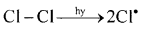Step 2: Propagation: Chlorine free radical reacts with methane to give methyl free radical.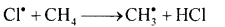The methyl free radical reacts with chlorine to form methyl chloride and chlorine free radical.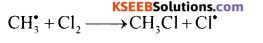Step 3: Termination: Free radials combine to form stable products.(b) Complete the following reaction.
(i) CaC2 + 2H2O → _____ + ______